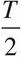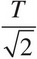# AP Physics 1 Question 251: Answer and Explanation

### Test Information

Question: 251

6. A simple pendulum swings about the vertical equilibrium position with a maximum angular displacement of 5° and period T. If the same pendulum is given a maximum angular displacement of 10°, then which of the following best gives the period of the oscillations?

• A.• B.• C. T
• D. 2T